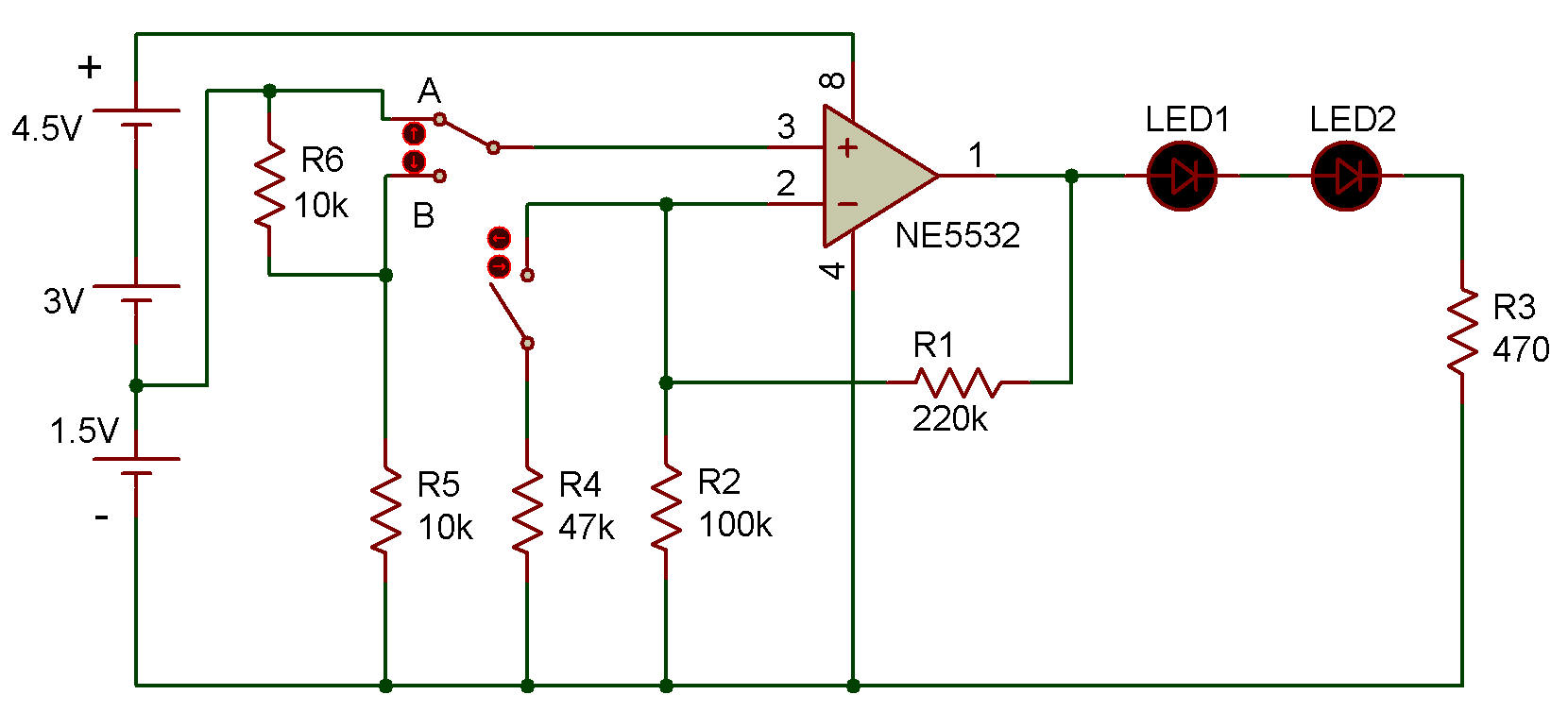Recherche personnalisé / personalised search

Basic Increase in DC Voltage

NE5532Now we move along to the simplest experiment on amplifying DC voltage. After you complete the wiring, set the switch to position B. LEDs 1 and 2 indicate the output voltage of the operational amplifier IC. An LED only lights when it is connected with about 1.5V. In this project we connect the two LEDs in series, so they only light when connected with about 3V. Now they are off, so the output voltage of the operational amplifier must be less than 3V.

Look at the schematic diagram. With the switch at position B, a 10K ohm resistor is connected in series between each of the battery terminals and the positive (+) input terminal of the operational amplifier. These two 10K ohm resistors divide the 1.5V supply voltage in half. This means the positive input terminal receives an input voltage of only 0.75V. To calculate the output voltage of the operational amplifier you multiply its input voltage by the amplification factor (R1/R2) + 1. So, the output voltage is 0.75V χ {(220Κ ohms / 100K ohms) + 1} = 2.4V.

Now, slide the switch to position A. This eliminates the 10K ohm resistors from the circuit, so the amplifier's positive input terminal receives the full 1.5V input voltage. Using the above equation, you can see that the output voltage of the operational amplifier is now 1.5V Χ {(220Κ ohms / 100K ohms) + 1} = 4.8V. The LEDs light dimly because the voltage supplied to them is more than3V. Let's change the amplification factor.

Slide the switch to position Β again and press the key. This adds the 47K ohm resistor to the 100K ohm resistor in parallel making total resistance of R2 about 32K ohms. (Do you remember how to calculate the total resistance for parallel connection from Project Resistors in Parallel in Resistor Section ). Now the output voltage is 0.75V χ {(220Κ ohms / 32K ohms) + 1} = 5.9V, enough to light the LEDs. If you slide the switch to position A again and press the key to connect 1.5V to the amplifier's positive ( + ) input terminal, the LEDs light brightly. Try to calculate the value of the output voltage with the switch at position A with the key pressed.

Recherche personnalisée